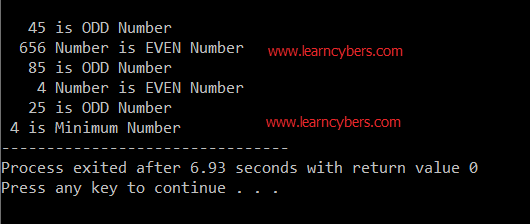To advertise with us contact on Whatsapp: +923041280395 For guest post email at: [email protected]

# Even odd program in C using for loop## Overview

The following source code is written in C Language which takes input from the user in an array, finds even, odd and minimum number in an array and prints these values on the screen.

## Explanation:

Line 1 imports built-in input and output library of C language. #include is a pre-processor in C programming and stdio.h is standard input output header. All the lines starting with // are comments. These lines are not executed while running program, however, these are added in code to enhance understanding of the code resulting in improved reusability.

### void getArray(int a[])

Line 4 defines a function of void type named getArray which takes 1D array as parameter. At line 7, counter variable i is initialized. At line 8, for loop is defined. All code inside block of for loop will be repeated until the value of i becomes less equal to 5. Line 12 prints prompt to ask user for entering number and line 14 invoke the scanf to get and store user input in array.

### void FindEven(int a[])

At line 22, variables are initialized. At line 24, for loop is defined. This loop will repeat the code inside it for 5 times from 0 to 4. At line 27, the c program finds the remainder. If the answer of remainder is 0, it means the entered number is even and completely divisible by 2, otherwise, it will be odd number.

### void FindEven(int a[])

This function iterate through each value of size 5 array. At line 40, it stores value at first index of array in min variable. After this, it starts looping through the array and compares each value of index+1 with value stored in min. At line 45, if value at a[i] (where a is array name and i is current index of array) is less than min, new minimum value is assigned to min variable.

### int main()

This function calls previously created functions to execute them.

## Source Code

```#include<stdio.h>

//function to get array input from user
void getArray (int a[])
{
//initialize variable
int i;
//for loop to get 5 values from user
for(i = 0; i < 5; i++)
{
//prompt message to ask user for input
printf("%2d-Enter any number : ", i+1);
//scan allows user input
scanf("%d", &a[i]);
}
}
//This function finds even and odd numbers from the numbers in array
//given by user
void FindEven(int a[])
{
//initialize variables
int i, j = 0;
//for loop definition
for(i = 0 ; i < 5 ; i++)
{
//condition to check if number is completely divisible by 2 or not and assign remainder to j variable
j = a[i] % 2;
//if number is completely divisible by 2, it will be even otherwise odd
if (j==0)
{
printf("\n%5d Number is EVEN Number", a[i] );
}
else printf("\n%5d is ODD Number", a[i]);
}
}
//This function finds minimum number in given array
void FindMin(int a[])
{
//initialization of variables
int i, min = a;
//for loop definition
for(i = 0 ; i < 5 ; i++)
{
//compares minimum value in each index of array
if (a[i] < min)
min = a[i];
}
printf("\n %d is Minimum Number", min);
}
//main method to call functions and pass values
int main()
{
int array, i;
getArray(array);
system("cls");
FindEven(array);
FindMin(array);
return 0;
}```

## Conclusion

We have successfully taken input from user in an array, checked each value in an array to find even and odd numbers, and identified the minimum number given by the user.